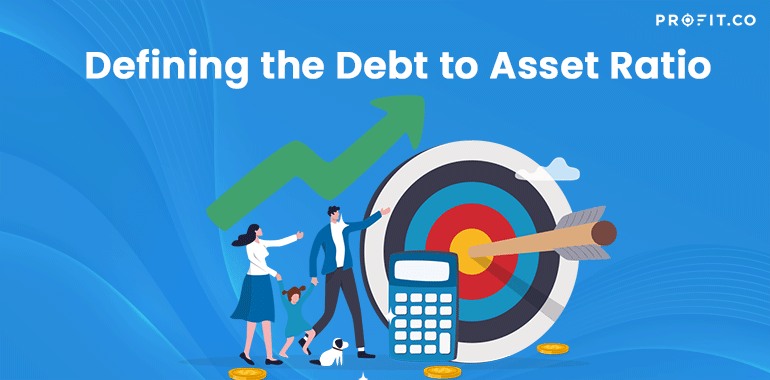Category: Finance KPIs.

To begin with, the debt to asset ratio could be defined as a leverage ratio, calculating the total amount of assets financed by creditors, as opposed to investors. That is to say, it indicates the percentage of assets that is funded by borrowing, in relation to the percentage of resources that are specifically funded by the investors. Essentially, it points out the way in which a company has grown and developed over time when it comes to acquiring assets.

Fundamentally, companies have the option of generating investor interest in an attempt to obtain capital and generate profit in order to acquire assets or take on debt.

The reason why this is such an important measurement is that it displays how leveraged a company is by assessing the number of resources owned by shareholders in the shape of equity and by creditors in the shape of debt. Thus, creditors and investors alike utilize this figure in order to make significant financial decisions.

In the position of an investor, you have to ensure that a company is solvent. In other words, it must have sufficient cash to address its existing financial obligations. At the same time, it should be successful enough to be financially capable of paying a return on investments.

On the other hand, creditors want to determine the amount of debt a company already has. That’s specifically because they want to find information concerning collateral and the firm’s financial capability of making repayments. In the case in which a firm has already leveraged all its assets and isn’t capable of addressing its monthly payments, a lender will be less likely to extend a line of credit.

The Formula of the Debt to Asset Ratio

The formula of the debt to asset ratio is calculated by dividing the total debt by the total assets. It is crystal clear that the equation is straightforward and simple. Its goal is to calculate the total debt as a given percentage of the total amount of assets. Essentially, there is more than one variation to this formula, which might include only specific assets or liabilities such as the current ratio.

Nevertheless, this particular financial comparison represents a global measurement that aims to assess a company as a whole.

Analyzing the Debt to Asset Ratio

Fundamentally, creditors, analysts and investors alike utilize this formula to see the overall risk level of a company. If the ratio is higher, then it is considered a risky investment, since it is more leveraged. That would mean that the company would have to pay out a notable percentage of its profits in interest and principle payments than a company with a lower ratio, which operates in the same industry. That being said, a lower ratio is more favorable.

On the other hand, if the debt to asset ratio is 1, that means the company has the same amount of assets and liabilities, being highly leveraged.

As you can see, the debt to asset ratio is an important financial tool for analysts, creditors, and investors for the reasons presented in this article. It is an essential ratio that outlines a firm’s overall risk level.

## Related Articles

• ### Sharpe Ratio

In order to be sound in your financial goals, you need to have a great source of knowledge on every... Read more